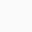## JS Reduce and forEachI have been polishing my Go and JS skills recently and today learned about how to use the reduce() and forEach() method. The problem I was trying to solve was return columns and rows from an input string.

A new line separator is used to define a new row and for each element is separated by ‘ ‘.

I started off with a basic class (code below)

```export class Matrix {
constructor(matrix) {}
}```

The matrix is the input string that we shall be using to populate rows and columns.

## Parsing

### Rows

```this.rows = matrix.split('\n')
.map(row => row.split(' ')
.map(r => +r));```

Parsing rows was relatively simple, I used the map method to find individual element and convert it to a number. (Numeric representation), if numeric is not required a simple split should do.

### Columns

```1.  this.columns = this.rows.reduce(
2.  (columns, row, i) => {
3.   row.forEach((current, j) => {
4.   if (i===0) {
5.    columns.push([row[j]]);
6.   } else {
7.    columns[j].push(current);
8.   }
9.  });
10.  return columns;
11. }, []);```

In row 1. I simply invoked reduce() method on rows which we parsed in the step above.

The reducer function takes four arguments:

1. Accumulator (acc)
2. Current Value (cur)
3. Current Index (idx)
4. Source Array (src)

Your reducer function’s returned value is assigned to the accumulator, whose value is remembered across each iteration throughout the array and ultimately becomes the final, single resulting value.

`(columns, row, i)`

We intent to return the columns which shall have the individual column read from the array of rows. Each ‘row’ will be representation of one such row [ ] and ‘i’ is the index.

`If there are 3 rows that were read. e.g. [0,1,2],[3,4,5],[5,6,7] the i will iterate through 0..2`

For each row that we get in step 2, we will iterate through the individual elements within that row to be added to column array (forEach).

forEach is taking two arguments

```(current, j)
Current -> Identifies the value being processed.
j       -> Identifies the index.```

The processing is done between line 4 – 8.

So if we consider an example

```    0 1 2
--------------
0 | 1 2 3 \n
1 | 4 5 6 \n
2 | 7 8 9 \n```

So the rows get populated as

```row = [
rows = [1, 2, 3]
rows = [4, 5, 6]
rows = [7, 8, 9]
]```

So, the processing of line 4 – 8

```row +> 1, 2 ,3 & i =0
The first iteration starts at
current = 1, j = 0

Line 4. since i = 0
columns = 1
The loop repeats
columns = 2
The loop repeats
columns  = 3
The outer loop repeats
row +> 4, 5 ,6 & i =1
current = 4, j = 0

& the same way the logic repeats.```

Finally you will have columns

`columns = [[1,4,7], [2, 5, 8], [3, 6, 9]]`

– THE – END –

## One thought on “JS Reduce and forEach”

1.Ranjana says:

Found other way to do the columns processing.

this._columns = Array.from({ length: this.rows.length}, (_,i)=> {
return this.rows.map((row) => row[i]);
});